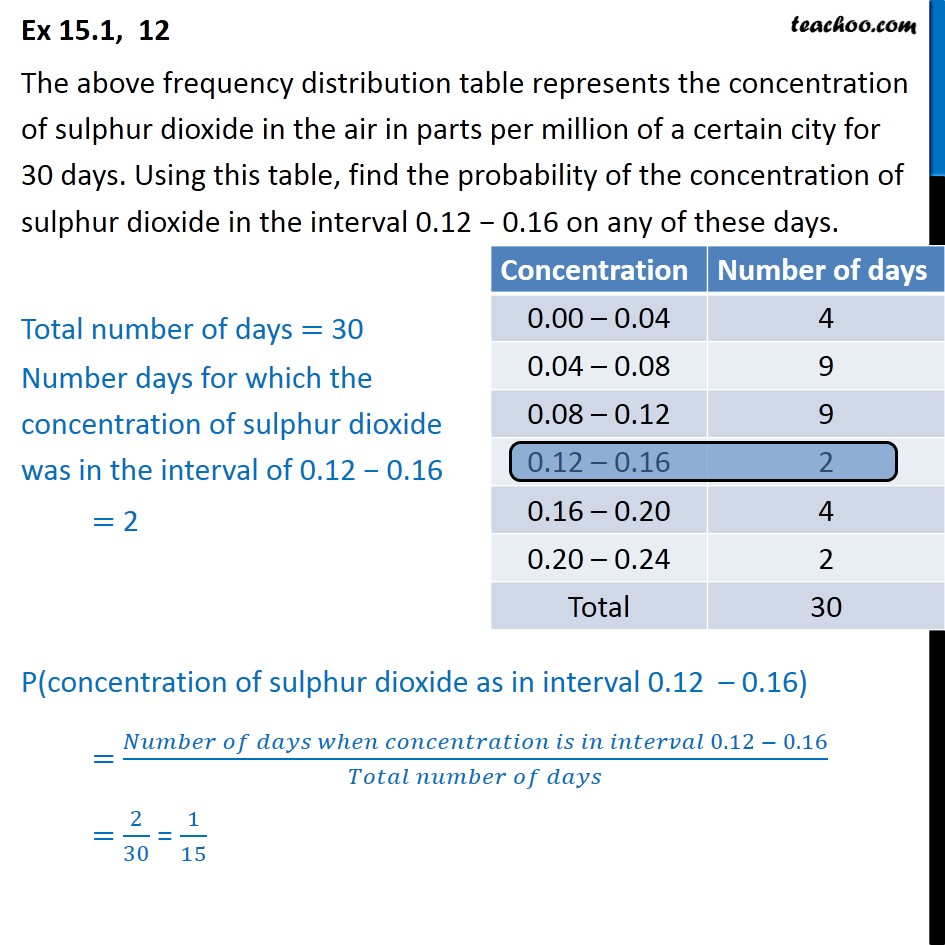1. Chapter 15 Class 9 Probability
2. Concept wise
3. Grouped frequency

Transcript

Ex 15.1, 12 The above frequency distribution table represents the concentration of sulphur dioxide in the air in parts per million of a certain city for 30 days. Using this table, find the probability of the concentration of sulphur dioxide in the interval 0.12 0.16 on any of these days. Total number of days = 30 Number days for which the concentration of sulphur dioxide was in the interval of 0.12 0.16 = 2 P(concentration of sulphur dioxide as in interval 0.12 0.16) = ( 0.12 0.16)/( ) = 2/30 = 1/15

Grouped frequency

About the AuthorDavneet Singh
Davneet Singh is a graduate from Indian Institute of Technology, Kanpur. He has been teaching from the past 10 years. He provides courses for Maths and Science at Teachoo.